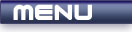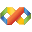Home Latest News Archive Tutorials C++ 2 3 4 Win32 Java OpenGL GLUT OpenGL ES 2 3 4 DirectX VB.NET HTML Photoshop 3dsmax Forums Projects Modelling Links About Us Contact Search Recent Tutorials C / C++ Win32 OpenGL OpenGL ES GLUT## Data Types

Tutorials > C++ > Data Types

### What are Data Types?

Data types are used to tell C / C++ what type of information you are working with eg. Are you working with a decimal number or are you working with a normal whole number.

### The different Data Types

Many data types can be shown in the table below :

 Data Type Data Size Description char 1 byte Single ascii character short 2 bytes Whole number from 32,768 to 32,767 int 4 bytes Larger whole number long 4 bytes Larger whole number float 4 bytes Decimal Number double 8 bytes Greater precision decimal number

### What are Variables?

Variables are used to store information temporarily while working with it in programs.

Variables are defined as follows :
data type variable name;
Variables may also be initialized with a particular value :
data type variable name = value;

Contents of main.cpp :

The limits.h file stores the INT_MAX and INT_MIN variables used in the program.

```#include <stdlib.h>
#include <limits.h>
#include <iostream>

using namespace std;

int main()
{
```

When giving a value for a float, you may need to add an f to the end of the number to prevent compiler warning. A plain decimal number in code is automatically seen as a double.

```	// Initialize Variables
char c = 'a';
short s = 56;
int i = 4500;
long l = 500000;
float f = 45.34f;
double d = 233423.2342;
```

The cout object allows you to easily output different data types. The sizeof function accepts one parameter which is any variable. It returns an int. It shows the amount of memory required for the variable.

```	// Output variable sizes
cout << "Char Size : " << sizeof(c) << " byte" << endl;
cout << "Short Size : " << sizeof(s) << " bytes" << endl;
cout << "Int Size : " << sizeof(i) << " bytes" << endl;
cout << "Long Size : " << sizeof(l) << " bytes" << endl;
cout << "Float Size : " << sizeof(f) << " bytes" << endl;
cout << "Double Size : " << sizeof(d) << " bytes" << endl;
cout << endl;
```

The code below shows different data types being displayed. You can also see how code can be placed on different lines. This is why the ; is so important as it specifies where the end of a code statement lies.

```	// Output variable values
cout << "Values : "
<< c << " "
<< s << " "
<< i << " "
<< l << " "
<< f << " "
<< d << endl;

cout << endl;
```

You may also have unsigned variables which may not contain negative values. You can see by using the code below that an unsigned variable can store a positive amount equal to double that of the original amount.

```	// Example of unsigned variables
unsigned int ui = 23;
cout
<< "Maximum Int : " << INT_MAX
<< " Minimum Int : " << INT_MIN << endl;

cout
<< "Max Unsigned : " << UINT_MAX
<< " Min Unsigned : " << 0 << endl;
```

A constant variable can also be created by using the const keyword in front of a data type. This value can never be changed and the compiler will complain if you try to change it. The INT_MAX and INT_MIN shown above are actually constant variables.

```	// Constants
const int noChange = 32;

system("pause");

return 0;
}
```

Congratulations. You should now be able to declare and define different types of variables and you should be able to output the amount of memory used by certain variables.

Please let me know of any comments you may have : Contact Me

 Source Files :< Tutorial 04 - Basic Output Tutorial 06 - Advanced Output >